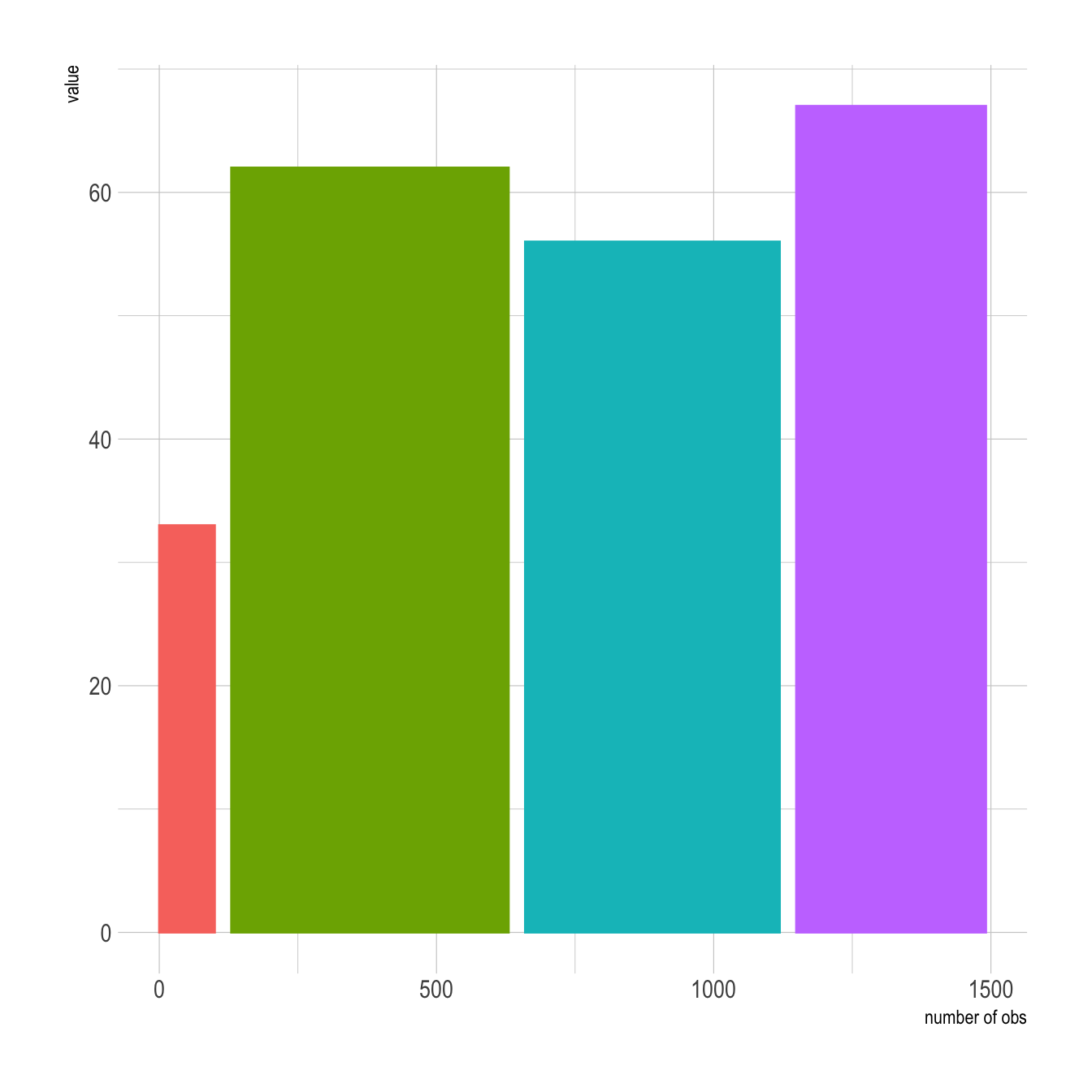# Barplot with variable width - ggplot2

This post explains how to draw a barplot with variable bar width using R and ggplot2. It can be useful to represent the sample size available behind each group.

This example shows how to customize bar width in your barchart. It can be used to show the sample size hidden between each category.

It is not possible to draw that kind of chart using `geom_bar()` directly. You need to compute manually the position of each bar extremity using the `cumsum()` function, and plot the result using `geom_rect()`.

Note: if you know what the distribution behind each bar is, don’t do a barplot, show it.``````# Load ggplot2
library(ggplot2)
library(hrbrthemes) # for style

# make data
data <- data.frame(
group=c("A ","B ","C ","D ") ,
value=c(33,62,56,67) ,
number_of_obs=c(100,500,459,342)
)

# Calculate the future positions on the x axis of each bar (left border, central position, right border)
data\$right <- cumsum(data\$number_of_obs) + 30*c(0:(nrow(data)-1))
data\$left <- data\$right - data\$number_of_obs

# Plot
ggplot(data, aes(ymin = 0)) +
geom_rect(aes(xmin = left, xmax = right, ymax = value, colour = group, fill = group)) +
xlab("number of obs") +
ylab("value") +
theme_ipsum() +
theme(legend.position="none") ``````

Related chart types

## Contact

This document is a work by Yan Holtz. Any feedback is highly encouraged. You can fill an issue on Github, drop me a message on Twitter, or send an email pasting yan.holtz.data with gmail.com.